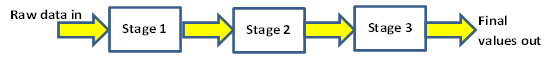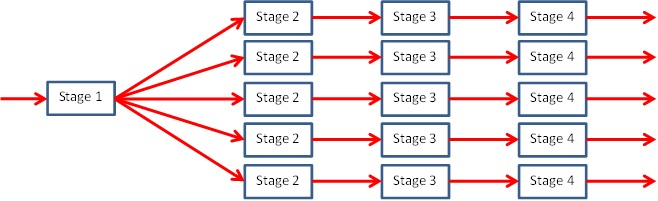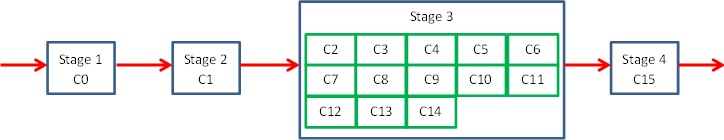# Pipelines on the Epiphany

In the previous tutorial (available here) we looked at splitting a problem up geometrically. Driven by the decomposition of the data, different parts of the problem ran on different Epiphany cores with these cores often needing to communuicate when a neighbouring value held on another core was required.
Whilst geometric decomposition is a very common approach not all problems are suited to being split around the geometry of the data and instead this tutorial we will look at splitting up a problem based upon the flow of data, known as a pipeline.

Before going any further, if you have not yet used or installed ePython then it is worth following the first tutorial (here) which walks you though installing ePython and running a simple “hello world” example on the Epiphany cores. If you installed ePython a while ago then it is worth ensuring that you are running the latest version, instructions for upgrading are available here.

## Pipelines

Data flows into the first stage, some processing is performed on it and a resulting value flows into the next stage, which performs processing and passes it onto the next stage etc.. Once data has been sent from one stage to the next then that stage is ready to receive some more data and start processing that.This is illustrated in the diagram, in a pipeline data only flows one way (here from left to right) and at each stage the data is refined, from its initial “raw” value to the final “finished” value. Ideally you want all stages in the pipeline to be busy, when your program starts it takes some time to fill up the pipeline and at the end the pipeline drains. The simplest approach to a pipeline will map a single stage to a single Epiphany core.

## ePython pipeline

Now it’s time for an example, based upon a large set of numbers we want to know the percentage of numbers that are contiguous, i.e. where the same numeric value lies one after another. This leads to a pipeline with four stages:

• Stage 1: Decide the number of data elements (chosen randomly) for that specific sequence.
• Stage 2: Based upon the number of elements generate random numbers for each of these.
• Stage 3: Sorts the number sequence
• Stage 4: Progresses through the sequence and counts the number of contiguous elements, the percentage of which is output

The input to the entire pipeline is the number of sequences to work on and the output of the pipeline is the percentage of contiguous numbers in that sequence.
```import parallel import util from random import randrange   data=*510   if (coreid()==0):   pipelineStageOne(100) elif (coreid()==1):   pipelineStageTwo() elif (coreid()==2):   pipelineStageThree() elif (coreid()==3):   pipelineStageFour()   def pipelineStageOne(num_items):   for i in range(num_items):     num=randrange(500) + 5     send(num, coreid()+1)   send(-1,coreid()+1)   def pipelineStageTwo():   num=0   while num >= 0:     num=recv(coreid()-1)     if num > 0:       i=0       while i < num:         data[i]=randrange(10)         i+=1       send(num, coreid()+1)       if num > 0: send(data, coreid()+1, num)   def pipelineStageThree():   num=0   while num >= 0:     num=recv(coreid()-1)     if num > 0:       data=recv(coreid()-1, num)       oddSort(data, num)     send(num, coreid()+1)     if num > 0: send(data, coreid()+1, num)   def pipelineStageFour():   num=0   num_contig=0.0   total_num=0   while num >= 0:     num=recv(coreid()-1)     if num > 0:       total_num+=num       data=recv(coreid()-1, num)       cnum=data       ccount=1       i=0       while i < num:         if (data[i] == cnum):           ccount+=1         else:           num_contig+=ccount           cnum=data[i]           ccount=0         i+=1     chance=(num_contig/total_num)*100     print chance+"% of numbers were contiguous" ```
This is an illustration of the code, the executable version is here
Based upon its core ID, a core will execute a specific pipeline stage function where it waits for data and, once it has received this, will process the data and send results onto the next stage. The oddSsort function (in the util module) will perform an odd-even sort on the number sequence. At the end of the pipeline, stage one will send the value -1 to stage two, which will then send it along to the next stage and quit. This action is repeated for the other stages and this is known a sentinal or poison pill, which will shut the pipeline down and this is the common way in which one terminates parallel pipelines.
So, we now have a pipeline which passes data between the stages and each stage operates on this data. However there is a problem, namely that the amount of work per pipeline stage is very uneven. For instance stage 1 will progress very quickly, whereas stage 3 (the sorting stage) will take much longer and fast stages will be held up by the slower stages. Bear in mind though, that we are only mapping one stage to one Epiphany core, so our current pipeline is only using 4 of the Epiphany cores. Hence we have 12 idle cores and how can we take advantage of these to help address our work imbalance problem and improve performance?

## Splitting the pipeline

What we are going to do here is keep stage 1 unique (i.e. on core 0), but then duplicate stages 2, 3 and 4 across all the remaining cores. This is known as a non-linear pipeline and it will look like the diagram here:Importantly this approach keeps all the cores busy and we have further parallelised the problem by adopting this splitting. Not only will each of the four stages operate in parallel, but also multiple cores will be performing the exact same stage work.
```..... if (coreid()==0):   pipelineStageOne(100) else:   if (coreid() % 3 == 1):     pipelineStageTwo()   elif (coreid() % 3 == 2):     pipelineStageThree()   else:     pipelineStageFour()   def pipelineStageOne(num_items):   matchingpid=1   for i in range(num_items):     num=randrange(500) + 5     send(num, matchingpid)     matchingpid+=3     if matchingpid > 13: matchingpid=1   for i in range(1,13,3):     send(-1,i)   ..... ```
This is an illustration of the code, the executable version is here
The code is very similar to the previous simple pipeline code, but stage 1 (on core 0) is maintaining a matching core ID, matchingpid which is sends to next. This value is increased at each stage and then wrapped around once matchingpid reaches over 13.
Time both the simple and split versions (using the -t command line argument for timing information.) You should see quite a significant performance improvement by adopting this splitting approach and taking advantage of the idle cores.

## Parallelising a specific stage

It is quite simple really, to improve performance we want to take advantage of the simple pipeline’s idle cores. As we have seen one way is by splitting and duplicating stages. The other way is by keeping the stages exactly the same, but instead to parallelise one specific stage. In our example the sorting (stage 3) is the most expensive, so we can concentrate our idle cores onto this stage.This is illustrated in the diagram, where *Cn* represents the *nth* Epiphany core and you can see that there are 13 cores allocated to stage three. This can work very well when another pattern can easily be adopted within the stage and here we are going to use geometric decomposition, to split the data up amongst these 13 cores and do a parallel sort on it.
```.....   if (coreid()==0):   pipelineStageOne(10) elif (coreid()==1):   pipelineStageTwo() elif (coreid() >= 2 and coreid() <= 14):   pipelineStageThree() elif (coreid()==15):   pipelineStageFour()   .....   def pipelineStageTwo():   num=0   while num >= 0:     num=recv(coreid()-1)     j=2     while j > =14:     if num > 0:       i=0       while i < num/13:         data[i]=randrange(1000)         i+=1       send(num/13, j)       send(data, j, num/13)     else:       send(-1, j)     j+=1   def pipelineStageThree():   num=0   while num >=0:     num=recv(1)     if num > 0:       data=recv(1, num)       parallel_odd_even_sort(num)     send(num, 15)     if num > 0: send(data, 15, num)   def pipelineStageFour():   data*100   num=0   num_contig=0.0   total_num=0   while num >= 0:     i=2     while i <= 14:       num=recv(i)       if (num > 0):         rdata=recv(i, num)         j=num*i         while j < num*(i+1):           data[j]=rdata[j - (num*i)]           j+=1       i+=1     if num > 0:       .....     chance=(num_contig/total_num)*100     print chance+"% of numbers were contiguous" ```
This is an illustration of the code, the executable version is here
This approach is a bit more complex as, instead of filling in the entire number sequence and passing it along, stage two of the pipeline will complete each subsequence needed for the different cores of stage three and send the specific data to its specific core. The Epiphany cores allocated to stage three then receive their subdata, perform a parallel sort on it (via the parallel_odd_even_sort function) and send their values onto stage four which will collate and assemble them in order to perform the final calculation.

## Summary

In this tutorial we have looked at pipelines where the parallelism is oriented around the flow of data. As it flows through the pipeline’s stages, data is refined until we get a final value that is output from the final stage. This approach is suited to many problems, and some that you might not nescesarily expect (such as CPU instruction pipelines.) Due to the fast interconnect between the Epiphany cores this approach of streaming data between them is potentially very advantageous – but as we have seen it is really important that each stage is equally busy at all times. If you have an uneven distribution of compution amongst the cores, or lots of idle cores, then splitting the pipeline or parallelising a specific stage can provide a significant gain.
More information about pipelines can be found here. An example focussing on the ePython sequential odd-even sort algorithm that we used can be found here and the parallel version we used can be found here.

### One Comment

• […] Sites Trusted Steroid Sites Trusted Steroid Sites Trusted Steroid Sites Trusted Steroid Sites Trusted Steroid Sites Trusted Steroid Sites Trusted Steroid Sites Trusted Steroid Sites Trusted Steroid Sites Trusted […]